Custom SearchIMPLICIT FUNCTIONS In equations containing x and y, separating the variables is not always easy. If we do not solve an equation for y, we call y an implicit function of x. In the equationy is an implicit function of x, and x is also called an implicit function of y. If we solve this equation for y, that is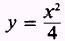then y would be called an explicit function of x. In many cases such a solution would be far too complicated to handle conveniently. When y is given by an equation such as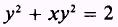y is an implicit function of x. Whenever we have an equation of this type in which y is an implicit function of x, we can differentiate the function in a straightforward manner. The derivative of each term containing y will be followed by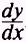. Refer to Theorem 6. EXAMPLE. Obtain the derivativeof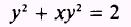SOL UTI0Y- Find the derivative of y2: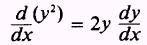the derivative of xy2: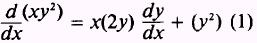and the derivative of 2: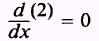such that,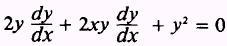Solving for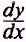we find that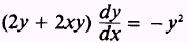andThus, whenever we differentiate an implicit function, the derivative will usually contain terms in both x and y. PRACTICE PROBLEMS: Find the derivativeof the following: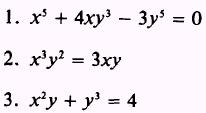ANSWERS: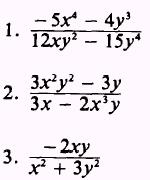Integrated Publishing, Inc. - A (SDVOSB) Service Disabled Veteran Owned Small Business